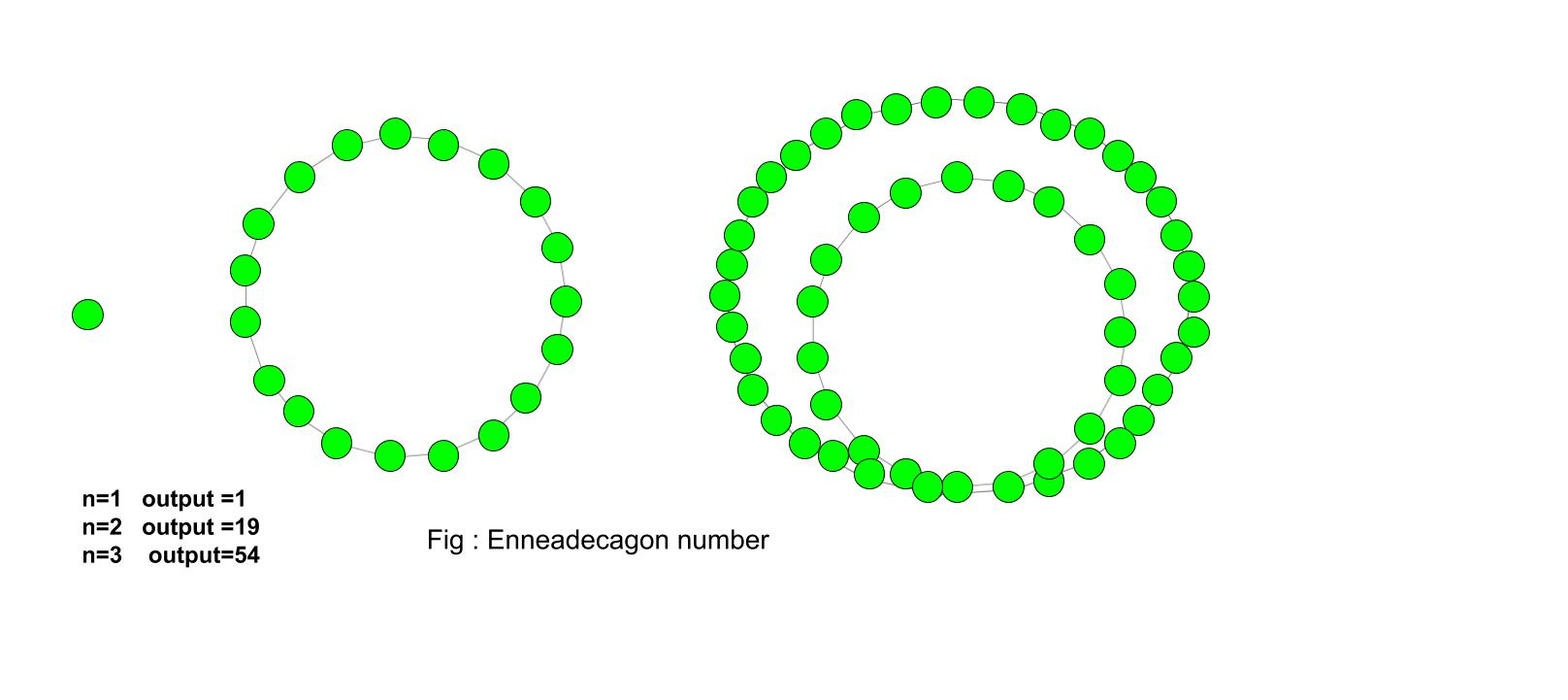Given a number n, the task is to find the nth Enneadecagonal number.
An Enneadecagonal number is a nineteen-sided polygon in mathematics. It belongs to a class of figurative number. The number contains the number of dots and the dots are arranged in a pattern or series. An Enneadecagonal number is also known as nonadecagon. The dots have common points and all other dots are arrange in the successive layer.

Examples :

Input : 4
Output :106

Input :10
Output :775Formula to find nth Enneadecagonal number :## C++

 // C++ program to find  // nth Enneadecagonal number  #include  using namespace std;     // Function to calculate   // Enneadecagonal number  int nthEnneadecagonal(long int n)  {      // Formula for finding      // nth Enneadecagonal number      return (17 * n * n - 15 * n) / 2;  }     // Drivers code  int main()  {      long int n = 6;      cout << n << "th Enneadecagonal number :" << nthEnneadecagonal(n);      return 0;  }

## Java

 // Java program to find  // nth Enneadecagonal number  import java.io.*;     class GFG {         // Function to calculate       // Enneadecagonal number      static int nthEnneadecagonal(int n)      {                     // Formula for finding          // nth Enneadecagonal number          return (17 * n * n - 15 * n) / 2;      }             // Driver Code      public static void main (String[] args)      {                     int n = 6;          System.out.print(n + "th Enneadecagonal number :");                 System.out.println( nthEnneadecagonal(n));      }  }     // This code is contributed by m_kit.

## Python3

 # Program to find nth  # Enneadecagonal number     def nthEnneadecagonal(n) :             # Formula to calculate nth      # Enneadecagonal number      return (17 * n * n - 15 * n) // 2    # Driver Code  if __name__ == '__main__' :                 n = 6     print(n,"th Enneadecagonal number :"                 , nthEnneadecagonal(n))     # This code is contributed  by Ajit

## C#

 // C# program to find  // nth Enneadecagonal number  using System;     class GFG  {      // Function to calculate       // Enneadecagonal number      static int nthEnneadecagonal(int n)      {                 // Formula for finding      // nth Enneadecagonal number      return (17 * n * n - 15 * n) / 2;      }             // Driver Code      static public void Main ()      {      int n = 6;      Console.Write(n + "th Enneadecagonal number :");             Console.WriteLine( nthEnneadecagonal(n));      }  }     // This code is contributed by aj_36

## PHP

 

Output :

6th Enneadecagonal number :261


Attention reader! Don’t stop learning now. Get hold of all the important DSA concepts with the DSA Self Paced Course at a student-friendly price and become industry ready.

My Personal Notes arrow_drop_upCheck out this Author's contributed articles.

If you like GeeksforGeeks and would like to contribute, you can also write an article using contribute.geeksforgeeks.org or mail your article to contribute@geeksforgeeks.org. See your article appearing on the GeeksforGeeks main page and help other Geeks.

Please Improve this article if you find anything incorrect by clicking on the "Improve Article" button below.

Improved By : jit_t